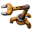Distortion noise function for a map mod

Place to post guides, observations, things related to modding that are not mods themselves.
Eketek
InserterPosts: 30
Joined: Mon Oct 19, 2015 9:04 pm
Contact:

Distortion noise function for a map mod

I was thinking about ways to improve map generation and possibly making a mod for it (the design I have in mind seems to go way beyond what it looks like Factorio supports for terrain auto-placement, so I'd probably have to generate every tile).

Part of this was thinking about noise algorithms and how to get more interesting results from a stateless generator. So, I applied a useful technique from sound synthesis: using functions to distort other functions (varying the input X & Y coordinates of a noise function by results taken from another). The results, in my opinion, are much more interesting than simply summing different octaves of coherent noise. I attached the results below. It's a fairly large image, so I also put it in a spoiler block.
Distorted noise - 80 test runs
I also have test code written in the Python programming language. It uses OpenSimplex to to generate noise and a fork of Python Imaging Library ("Pillow") to display results. Install those libraries, copy & paste the code into a python script (with python set to run as an intaractive shell). 'test()' should produce distorted noise with decent random parameters. The other more complicated function is more controllable.

Code: Select all

``````from PIL import Image
from random import randint
from random import random as rand
from math import sin
from math import cos
from math import pi as PI
from opensimplex import OpenSimplex

# Range of OpenSimplex appears to be [0 +- sqrt(.75)].  For obvious reasons, I prefer [-1:1].
NOISE_NORM_FACTOR = 1 / .75**.5

# Run a randomized test using some OK-ish parameters
def test(scale=4/512, size=512, ofs=None, seed_s=None, seed_a=None, seed_b=None):
if seed_s == None:
seed_s = randint(-2**31, 2**31)
if seed_a == None:
seed_a = randint(-2**31, 2**31)
if seed_b == None:
seed_b = randint(-2**31, 2**31)

s = OpenSimplex(seed_s)
a = OpenSimplex(seed_a)
b = OpenSimplex(seed_b)

res_ang = rand()**2 * 10

if ofs == None:
ofs = (randint(-2**31, 2**31),randint(-2**31, 2**31))

# Run the more sophisticated noise function with random parameters
def test2(scale=4/512, size=512, ofs=None, seed_s=None, seed_a=None, seed_b=None, seed_r=None):
if seed_s == None:
seed_s = randint(-2**31, 2**31)
if seed_a == None:
seed_a = randint(-2**31, 2**31)
if seed_b == None:
seed_b = randint(-2**31, 2**31)
if seed_r == None:
seed_r = randint(-2**31, 2**31)

s = OpenSimplex(seed_s)
a = OpenSimplex(seed_a)
b = OpenSimplex(seed_b)
r = OpenSimplex(seed_r)

res_ang = rand()**2 * 10
res_rel = rand()**2 * 10
#res_rel = 1
rd = rand() * 16
#rd = 0

if ofs == None:
ofs = (randint(-2**31, 2**31),randint(-2**31, 2**31))
print("res_ang: " + str(res_ang))
print("res_rel: " + str(res_rel))
print("rd: " + str(rd))
print("ofs: " + str(ofs))

# Distort a noise function by deriving displacement vectors from a pair of other noise functions
w = 0
h = 0
if type(size) is int:
w = size
h = size
else:
w = size
h = size
buf =  * (3*w*h)
for x in range(0,w):
for y in range(0,h):
_x = x+ofs
_y = y+ofs
angle = gen_angle.noise2d(_x*res_angle*scale_main, _y*res_angle*scale_main) * NOISE_NORM_FACTOR * PI

v = (int)((gen_out.noise2d(_x*scale_main + xD, _y*scale_main + yD) * NOISE_NORM_FACTOR + 1) * 128)

if v < 0:
v = 0
elif v > 255:
v = 255
buf[3 * (x + y*w) + 2] = v

img = Image.frombytes('HSV', (w,h), bytes(buf))
if f != None:
_img = img.convert('RGB')
_img.save(f)
_img.close()
else:
img.show()
img.close()

# Similar to preceding function, but with the length of the displacement vector scaled by another noise function
w = 0
h = 0
if type(size) is int:
w = size
h = size
else:
w = size
h = size
buf =  * (3*w*h)
for x in range(0,w):
for y in range(0,h):
_x = x+ofs
_y = y+ofs

angle = gen_angle.noise2d(_x*res_angle*scale_main, _y*res_angle*scale_main) * NOISE_NORM_FACTOR * PI

v = (int)((gen_out.noise2d(_x*scale_main + xD, _y*scale_main + yD) * NOISE_NORM_FACTOR + 1) * 128)

if v < 0:
v = 0
elif v > 255:
v = 255
buf[3 * (x + y*w) + 2] = v

img = Image.frombytes('HSV', (w,h), bytes(buf))
if f != None:
_img = img.convert('RGB')
_img.save(f)
_img.close()
else:
img.show()
img.close()``````

To actually integrate it into a mod for use as a terrain generator, my inclination would be to implement a 2d modular synthesizer (which outputs tile & resource generation commands instead of sound), to allow for excessive flexibility. That said, I haven't actually decided to make the mod, but I want to put the idea out there in case anyone with a bit more time wants to pick it up.

EDIT: Added an extra function which takes the concept a bit farther by deriving displacement vector lengths from another noise function (with significantly more varied results), and a corresponding test function to play with it, "test2()".

Who is online

Users browsing this forum: No registered users